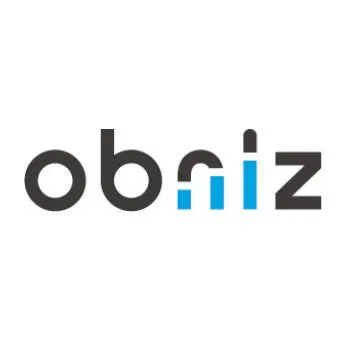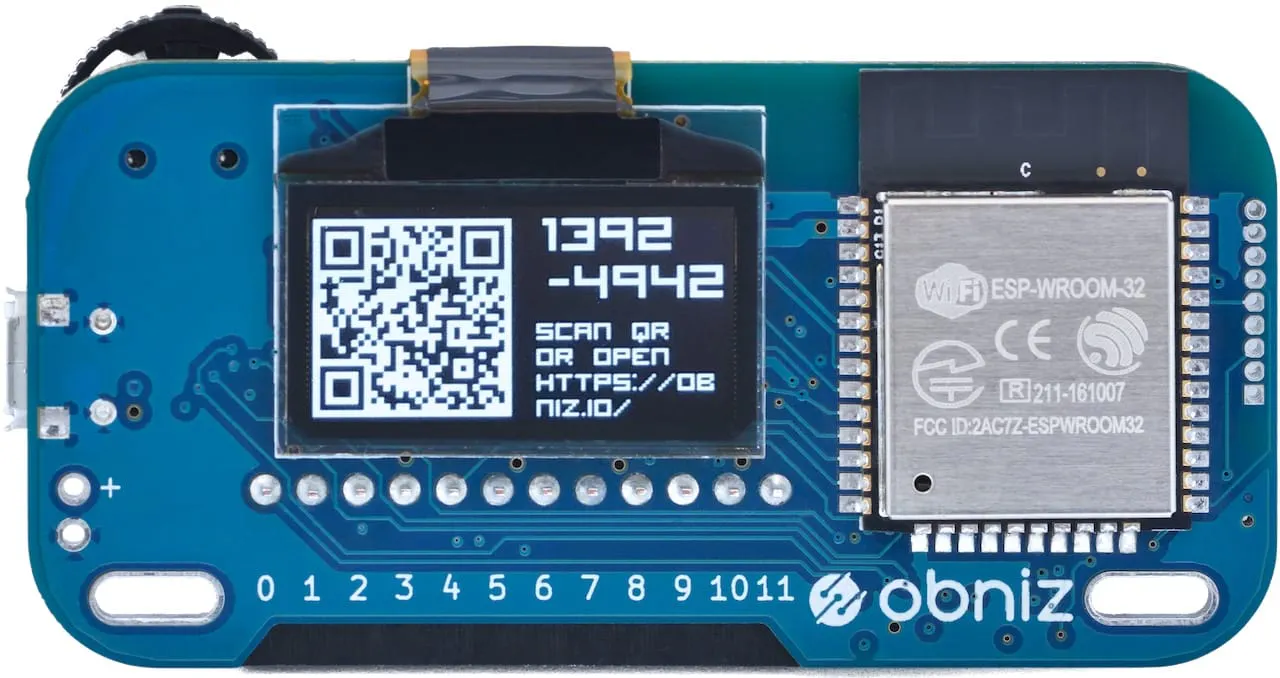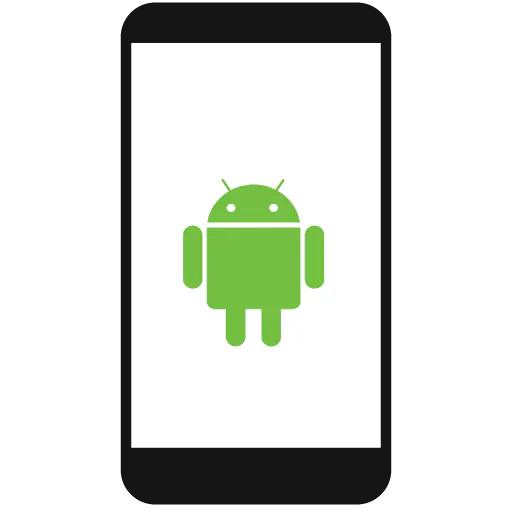# Obstacle Avoidance Game with Distance Sensor

Obstacle avoidance game like Flappy Bird. Move your hand to avoid the collision. It is easy to make and fun to play!

BeginnerFull instructions provided4 hours894

## Things used in this project

### Hardware componentsCambrian Robotics obniz obniz.io
×1
×1Apple iPhone Computer or smartphone
×1Android device Computer or smartphone
×1

## Code

### Program

JavaScript
```<html>
<meta name="viewport" content="width=device-width, initial-scale=1">
<script src="https://obniz.io/js/jquery-3.2.1.min.js"></script>
<script src="https://unpkg.com/obniz@1.9.1/obniz.js" crossorigin="anonymous"></script>
<body>

<div id="obniz-debug"></div>

<canvas id="field" width="300" height="300"/>

<script>
let canvas = document.getElementById('field');
let ctx = canvas.getContext('2d');
let dot = [];
let bars = [];
let inputHeight = 0;
let frameCount = 0;
let obniz = new Obniz("OBNIZ_ID_HERE");
obniz.onconnect = async function () {
let sensor = obniz.wired("GP2Y0A21YK0F", {vcc: 2, gnd: 1, signal: 0});
sensor.start(function (height) {
inputHeight = height;
})

};

function initialize() {
ctx.fillStyle = "black";
ctx.fillRect(0, 0, canvas.width, canvas.height);

ctx.fillStyle = "white";
ctx.font = "25pt Arial";
ctx.fillText("Click to START", 40, 150);

}

initialize();

function startGame() {

frameCount = 0;
for (let i = 0; i < canvas.width / 4; i++) {
dot[i] = undefined;
}
dot[dot.length] = canvas.height / 2;

bars = [];

frame();

}

function frame() {

frameCount++;
ctx.fillStyle = "black";
ctx.fillRect(0, 0, canvas.width, canvas.height);

let input = (300 - inputHeight);
input = Math.min(Math.max(0, input), canvas.height);
dot.push(input);
dot.shift();

for (let i = bars.length - 1; i >= 0; i--) {
bars[i].x--;
if (bars[i].x + bars[i].width < 0) {
bars.splice(i, 1);
}
}
if (frameCount === 100) {
frameCount = 0;
if (Math.random() > 0.3) {

bars.push({x: canvas.width, y: Math.random() * 380 - 80, width: 16, height: 160});
} else {
let hole = Math.random() * 300;
bars.push({x: canvas.width, y: 0, width: 16, height: hole - 40});
bars.push({x: canvas.width, y: hole + 40, width: 16, height: canvas.height});
}

}

drawDots();
drawBars();
if (isHit()) {
gameOver();
} else {
setTimeout(frame, 10);
}

}

function drawDots() {
ctx.strokeStyle = "white";
ctx.lineWidth = 1;
ctx.beginPath();

for (let i = 0; i < dot.length - 1; i++) {

if (dot[i] !== undefined && dot[i + 1] !== undefined) {

ctx.moveTo(i, dot[i]);
ctx.lineTo(i + 1, dot[i + 1]);
}
}
ctx.closePath();
ctx.stroke();

}

function drawBars() {
ctx.fillStyle = "red";
ctx.beginPath();

for (let i = 0; i < bars.length; i++) {

ctx.fillRect(bars[i].x, bars[i].y, bars[i].width, bars[i].height);
}

}

function isHit() {
let x = dot.length;
let y = dot[dot.length - 1];

for (let i = 0; i < bars.length; i++) {
if (bars[i].x <= x && x <= bars[i].x + bars[i].width
&& bars[i].y <= y && y <= bars[i].y + bars[i].height) {
return true;
}
}
return false;

}

function gameOver() {

ctx.fillStyle = "white";
ctx.font = "25pt Arial";
ctx.fillText("GAME OVER", 50, 100);
ctx.fillText("Click to START", 40, 200);

}

</script>
</body>
</html>
```

## Credits

### obniz developer team

59 projects • 24 followers
Development board "obniz" is controlled via the internet.